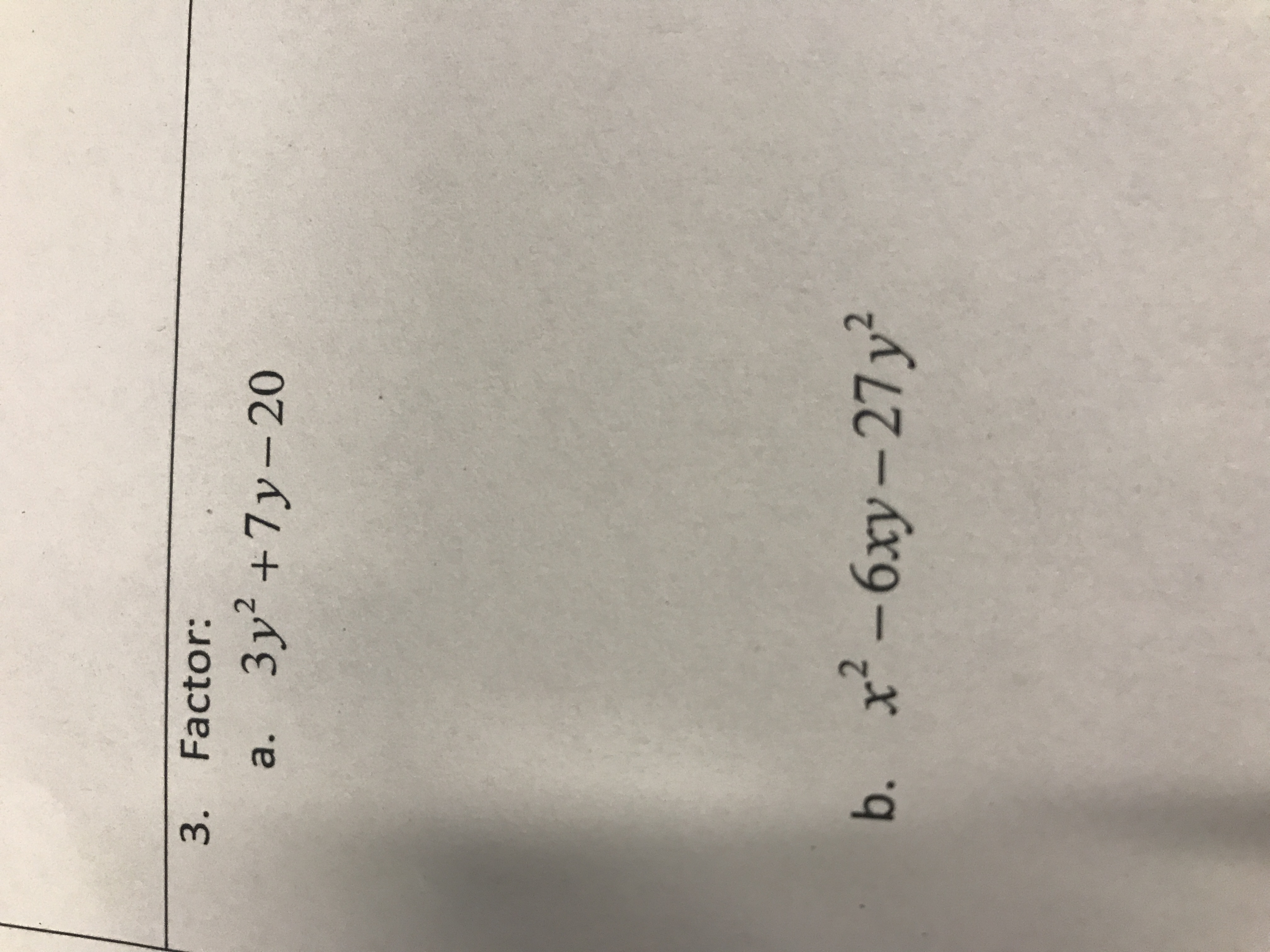3. Factor:3 y2 +7y-20Зу?a.b. x- 6ху- 27у*

Questionhelp_outlineImage Transcriptionclose3. Factor: 3 y2 +7y-20 Зу? a. b. x- 6ху- 27у* fullscreen
Step 1

First multiply 3 and -20. So -60.

Then we have to factor -60 so that sum of the factors =7

-60= -5 times 12

So we will write 7y=-5y+12y

Step 2

Then we factor by grouping.

Step 3

We have to multiply 1 and -27. So -27

Then we factor -27 so that sum of the factor...

Want to see the full answer?

See Solution

Want to see this answer and more?

Our solutions are written by experts, many with advanced degrees, and available 24/7

See Solution
Tagged in

Algebra